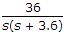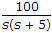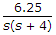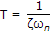# Electronics and Communication Engineering - Automatic Control Systems - Discussion

Discussion Forum : Automatic Control Systems - Section 1 (Q.No. 26)
26.
Consider the systems with following open loop transfer functions
1.2.3.If unity feedback is used, the increasing order of time taken for unit step response to settle is
1, 2, 3
3, 1, 2
2, 3, 1
3, 2, 1
Explanation:. For the three systems the values of ξωn are 1.8, 2.5 and 2 respectively.

Hence values of T are 0.55, 0.4 and 0.5 respectively.

Discussion:
3 comments Page 1 of 1.

Anish kumar said:   8 years ago
See.

100/s(s+5) open loop transfer function.

100/s(s+5)/(1+100/s(s+5) ) = 100/s2+5s+100.

So coefficient of s = 2*si*wn = 5.
= si*wn=2.5.

Settling time = 4/2.5 = 1.6.

Likewise for 1 t settling will be 2.2, for 2 t settling will be 1.6, and for 3 t settling will be 2.

So(1) = 2.2.
(2) = 1.6.
(3) = 2.5.

So increasing order will be 2, 3, 1.

Arslan said:   8 years ago
@Hina khan.

Kindly explain it again. I did not understand.

Hina khan said:   9 years ago
Settling time will be 4T so increasing order of Ts will be 2, 3, 1.